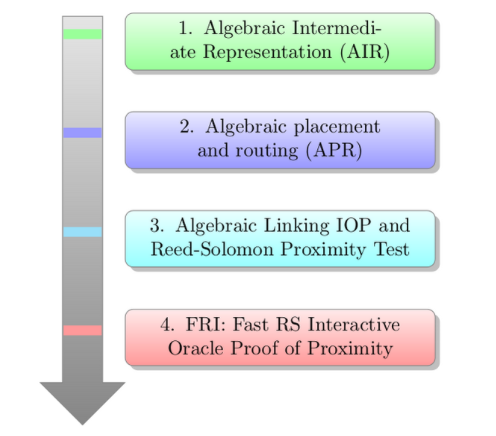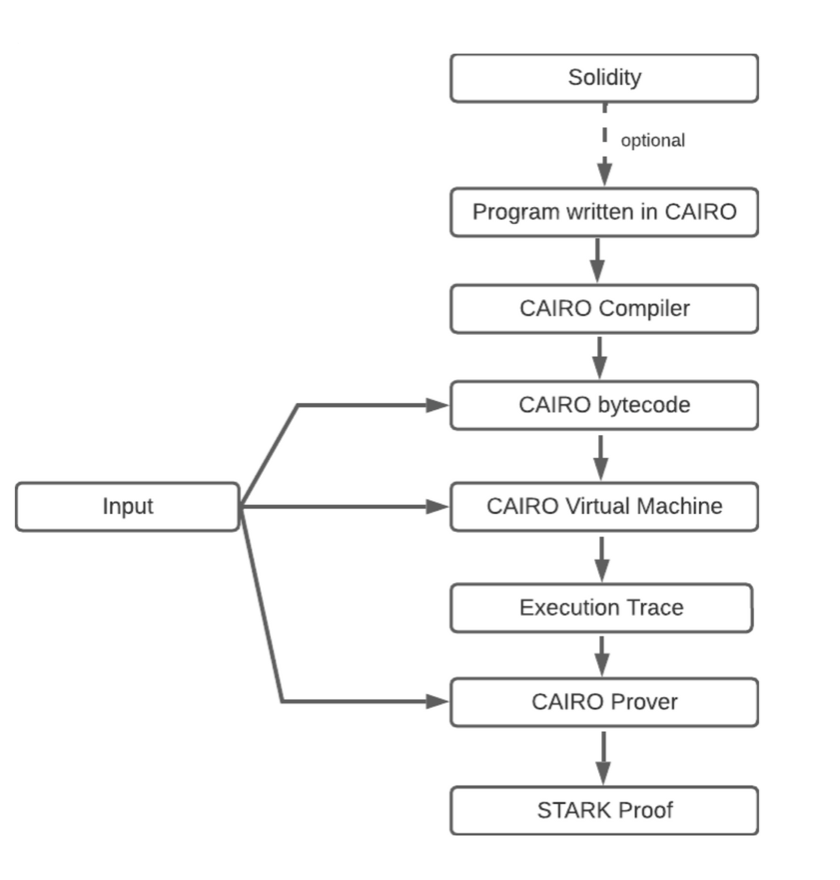# Introduction

Cairo is one of the smart contract languages for creating STARK-provable programs for general computation. It is developed by Starkware, for their permission-less decentralized* Validity-Rollup. The white paper can be found here. In this article, we discuss broader aspects of CAIRO.

To address the issue of scalability on Ethereum, (Layer-1) and Layer-2(L2) solutions were introduced. One most common kind of Layer-2(L2) solution is zk-rollups, which bundle off-chain L2 transactions and submit them as one transaction on Layer-1(L1). These systems use validity proofs to check the integrity of transactions and smart contracts. One of the ways to provide validity proofs is using zk-STARK.

# ZK-STARK

The term “zk-STARK” stands for “zero-knowledge Scalable Transparent ARgument of Knowledge”. Computations and storage can be done off-chain, and then the prover generates STARK proofs for computational integrity and then be placed on the chain for verification by a verifier. The time required by the verifier is then really small, this gives scalability. No trusted third party is required, hence it is transparent. A computation integrity check is reduced to a set of steps of integrity checks, each step connecting rows of a certain matrix, the latter is called execution trace. These checks are defined in terms of multivariate polynomials. This polynomial form is called an algebraic intermediate representation (AIR) of that computational integrity statement. Read more here, arithmetization. As a result of a polynomial expression, Expressing computational integrity in terms of polynomials allows for probabilistic testing to be performed, a key step in generating a STARK. This testing is to check whether the polynomials are of a low degree. And this test is done using FRI protocol, Fast Reed-Solomon Interactive Oracle Proof of Proximity. Here Fast comes from Fast-Fourier-transform style recursion, which is used to test proximity to a low degree polynomial by probabilistic testing.

The flow of the diagram looks as follows.Figure 1: Flow diagram for zk-STARK

In this approach, the proving/verifying programs depend on the computation. This results in two significant problems: a) Every new computation needs a new AIR program. This would mean different smart contracts would need different AIR programs. b) A verifier must update the verifying program, based on the new AIR program, which needs communication.

To avoid this, we need to implement any SMART contract or program logic with one AIR program

This is where CAIRO comes in.

# CAIRO

Cairo is a Turing complete language that stacks together smaller AIR and combines them into one AIR. The smaller AIR can be thought of as a single computation and multiple AIR represents arbitrary computation and thus solves the above problem, being able to implement any smart contract and also, having one AIR program. This has a CPU von Neumann architecture. The name CAIRO comes from CPU-AIR.

It has a register-based memory model and a compiler. The compiler produces a table of computational steps called a trace. The trace is used by the prover to construct AIRs which are combined, subjected to FRI testing, and then converted into a STARK proof.

It has the following components:

• The Cairo language: To write your programs/computations in a high-level functional language.
• The Cairo compiler: To translate your high-level code into bytecode (CPU instructions).
• The Cairo VM: execute the above bytecode and get an execution trace.
• The SHARP prover performs low-degree testing(FRI), generates a proof and Merkle tree that commits to this proof, and saves the commitments as CALLDATA in the verifier smart contract.
• A Cairo verifier: then verify a Cairo proof.Figure 2: CAIRO proof generation flow

The scalability can be measured in terms of complexity for the prover and verifier. If T is the number of steps of the AIR program, then

a) Proving time is quasi-linear in T, that is, it is Tpolylog(T)

b)Verification time is log(T)

# CAIRO CPU

We leave the complete description of CAIRO VM, which interested readers can read here. We describe the CAIRO CPU. The computation model is based on a CPU with three registers, each of which points to locations in the read-only memory. These are:

• The program counter(pc): (via the memory function) points to an address in memory from where to fetch the next instruction.
• Allocation pointer(ac): contiguous, points to a free address in memory, useful to store data globally.
• Frame pointer(fc): used to store data and do function calls and points to the function’s local memory. When a function is called, fc is set equal to ac, when that function returns, fc is restored to the value before the previous call.

The register and the memory values are elements of a finite field F, chosen to be of characteristic \$p =²²⁵¹+17•²¹⁹²+1. The following describes the machine instructions.

Cairo Machine instructions

a) Basic

• Assert statements two things are equal
• Conditional and unconditional jumps
• Call and return
• increment the allocation pointer.

b) Builtins:

• Predefined computations are useful in transactions like range check, hash(Pedersen), signatures (ECDSA), etc. which might be costly to perform using the basic instructions.
• Implicit arguments: some program calls are so common, that CAIRO has an implicit call to it.

Also, there are blocks of Python code, called hints, which the prover can execute right before the next instruction. These hints of unconditional jumps give a powerful tool of non-determinism to CAIRO.

# An example

CAIRO has an online program editor at https://www.cairo-lang.org/playground/, where you can run the program and also see the evolution of the registers and memory. An example of a function is here. This program verifies that the 3rd Fibonacci number is 5. Note [x] denotes the value of memory stored at the address [x].

`func main():    # Call fib(1, 1, 3).    [ap] = 1; ap++    [ap] = 1; ap++    [ap] = 3; ap++    call fib# Make sure the 3rd Fibonacci number is 5.    [ap - 1] = 5    retendfunc fib(first_element, second_element, n) -> (res : felt):    jmp fib_body if n != 0    [ap] = second_element; ap++    retfib_body:    [ap] = second_element; ap++    [ap] = first_element + second_element; ap++    [ap] = n - 1; ap++    call fib    retend`

Since its launch, STARKNET ecosystem is fast growing in areas of DeFi, NFTs, on-chain gaming, etc. An excellent source of information is here, from where the below picture is sourced.

# Concluding observations

In this article, we gave a bird-eye view of zk STARK, Virtual machine, and CAIRO. In CAIRO at the moment, (SHARP) SHARed Prover is still centralized and is run by STARKNET, although there is a discussion about decentralization using STARKNet tokens, more information here. STARKNET is not EVM-compatible, but one can use WARP to compile Solidity source code to custom ZK-friendly bytecode, along with writing contracts directly in CAIRO.

Compared to zk SNARKs, zk-STARK is more expensive in terms of gas fees, and the finality time is a lot more(2 hours vs 2–3 minutes). There is more work needed in that direction, particularly in the recursive STARKs.

On other hand, zk-STARK is quantum-resistant since it only depends only on hash functions, unlike SNARK which depends on pairings (and Discrete log on elliptic curves), and will be “vulnerable” if and when a reasonable quantum computer is built.

--

--